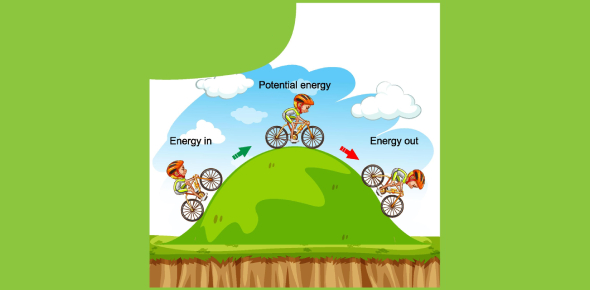# Quiz: Test Your Knowledge On Energy!

10 Questions | Attempts: 45630SettingsTest your knowledge on energy with the below quiz that we have brought here for you. A simple definition of energy is, it is the capacity to do work, move an object by some application of force, or heat an object. Energy is a quantitative property that can be transferred from objects to objects and can take forms but cannot be created or destroyed. This quiz will ask you some essential points about the topic. Let's see how well you know.

• 1.
Which of the following is a conversion from chemical energy to thermal energy?
• A.

Coal is burned at a power plant.

• B.

Gasoline is burned in a car engine.

• C.

Charcoal is burned in a barbeque pit.

• D.

All of the above.

• 2.
Which of the following is the best example of increasing an object's potential energy?
• A.

Rolling a bowling ball.

• B.

Turning on a light bulb.

• C.

Stretching a rubber band.

• D.

Dropping a pencil.

• 3.
An object that has kinetic energy must be...
• A.

Lifted above the earth's surface.

• B.

In motion

• C.

At rest

• D.

None of the above

• 4.
Thermal energy is...
• A.

Kinetic

• B.

Potential

• C.

Both kinetic and potential.

• D.

Neither kinetic nor potential.

• 5.
Sound energy is...
• A.

The energy of a compound that changes as its atoms are rearranged to form new compounds.

• B.

The total energy of the particles that make up an object.

• C.

The energy caused by an object's vibrations.

• D.

The energy of motion.

• 6.
What device converts chemical energy to mechanical energy?
• A.

Human

• B.

Car

• C.

Jet ski

• D.

All of the above.

• 7.
As height increases, so does...
• A.

Thermal energy.

• B.

Mechanical energy.

• C.

Kinetic energy.

• D.

Potential energy.

• 8.
The law of _________________ of energy states that energy cannot be created or destroyed.
• A.

Transformation

• B.

Absorption

• C.

Conservation

• D.

Atability

• 9.
According to the law of conservation of energy, in theory, a bouncy ball should never stop bouncing. However, we know that it eventually stops. Where does the energy go?
• A.

Some get converted into sound energy and escape into the surroundings.

• B.

Some get converted into thermal energy and escape into the surroundings.

• C.

Both A and B

• D.

None

• 10.
Energy is...
• A.

When the surfaces of two objects rub against each other

• B.

The ability to do work

• C.

Both A & B

• D.

None

## Related TopicsBack to top
×

Wait!
Here's an interesting quiz for you.